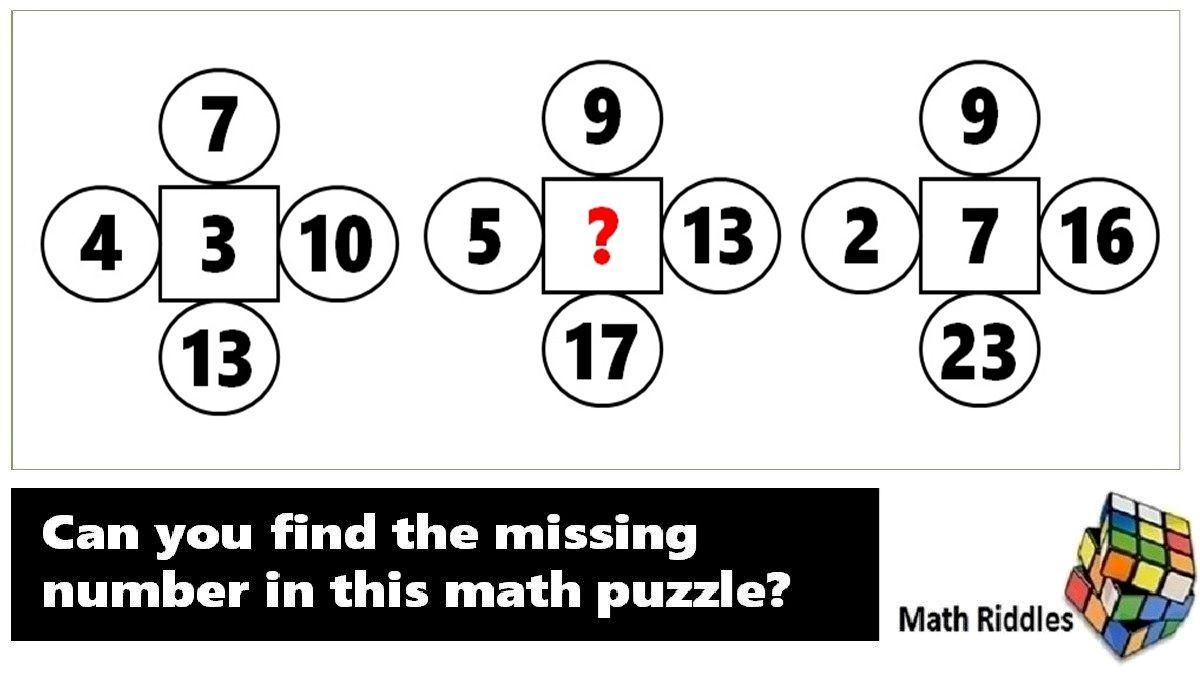# Math Riddles: Test Your IQ, Find the Missing Number Puzzles (with Answers)

Math Riddles Challenge: Can you find the missing numbers in these math puzzles? Check Your IQ.Math Riddles: Test Your IQ, Find the Missing Number Puzzles (with Answers)

Math Riddles with Answers: Do you enjoy Math Riddles? Do you love solving difficult math problems? Do you know how to solve number patterns? Here is another Math Riddle where you can put on your analytical thinking cap to find the missing numbers in these number series puzzles.

## Solution

### Logic Puzzle #1

Explanation

We will first solve Shape 1 and Shape 2 to understand the logic.

Shape 1

=> (12 + 4) x (8 – 5)

=> 16 x 3

=> 48

Shape 2

=> (15 + 4) x (11 - 5)

=> 19 x 6

=> 114

Similarly, we shall apply the same logic to solve Shape 3.

Shape 3

=> (18 + 3) x (18 - 12)

=> 21 x 6

=> 126

Hence, the missing number in Shape 3 is 126.

### Logic Puzzle #2

Explanation

We will start by solving Circle 1 and Circle 2 to figure out the logic.

Circle 1

=> (7 x 8) – (7 + 8) = 41

Circle 2

Moving on, we solve this circle with the same logic.

=> (5 x 9) – (5 + 9) = 31

Similarly, we shall apply the same logic to find out the missing number in the place of the question mark.

Circle 3

=> (11 x 7) – (11 + 7)

=> 59

## Tell us in comments: Did you solve these math puzzles in 20 seconds each?

Check out more math puzzles!